# Background subtraction – OpenCV

• Difficulty Level : Medium
• Last Updated : 19 Feb, 2020

Background subtraction is a way of eliminating the background from image. To achieve this we extract the moving foreground from the static background.

Background Subtraction has several use cases in everyday life, It is being used for object segmentation, security enhancement, pedestrian tracking, counting the number of visitors, number of vehicles in traffic etc. It is able to learn and identify the foreground mask.

In OpenCV we have 3 algorithms to do this operation –

BackgroundSubtractorMOG – It is a Gaussian Mixture-based Background/Foreground Segmentation Algorithm.

BackgroundSubtractorMOG2 – It is also a Gaussian Mixture-based Background/Foreground Segmentation Algorithm. It provides better adaptability to varying scenes due illumination changes etc.

BackgroundSubtractorGMG – This algorithm combines statistical background image estimation and per-pixel Bayesian segmentation.

How to apply OpenCV in-built functions for background subtraction –
Step #1 – Create an object to signify the algorithm we are using for background subtraction.
Step #2 – Apply `backgroundsubtractor.apply()` function on image.

Below is the Python implementation for Background subtraction –

 `# importing libraries ` `import` `numpy as np ` `import` `cv2 ` ` `  `# creating object ` `fgbg1 ``=` `cv2.bgsegm.createBackgroundSubtractorMOG();    ` `fgbg2 ``=` `cv2.createBackgroundSubtractorMOG2(); ` `fgbg3 ``=` `cv2.bgsegm.createBackgroundSubtractorGMG(); ` ` `  `# capture frames from a camera  ` `cap ``=` `cv2.VideoCapture(``0``); ` `while``(``1``): ` `    ``# read frames ` `    ``ret, img ``=` `cap.read(); ` `     `  `    ``# apply mask for background subtraction ` `    ``fgmask1 ``=` `fgbg1.``apply``(img); ` `    ``fgmask2 ``=` `fgbg2.``apply``(img); ` `    ``fgmask3 ``=` `fgbg3.``apply``(img); ` `     `  `    ``cv2.imshow(``'Original'``, img); ` `    ``cv2.imshow(``'MOG'``, fgmask1); ` `    ``cv2.imshow(``'MOG2'``, fgmask2); ` `    ``cv2.imshow(``'GMG'``, fgmask3); ` `    ``k ``=` `cv2.waitKey(``30``) & ``0xff``; ` `    ``if` `k ``=``=` `27``: ` `        ``break``; ` ` `  `cap.release(); ` `cv2.destroyAllWindows(); `

Output: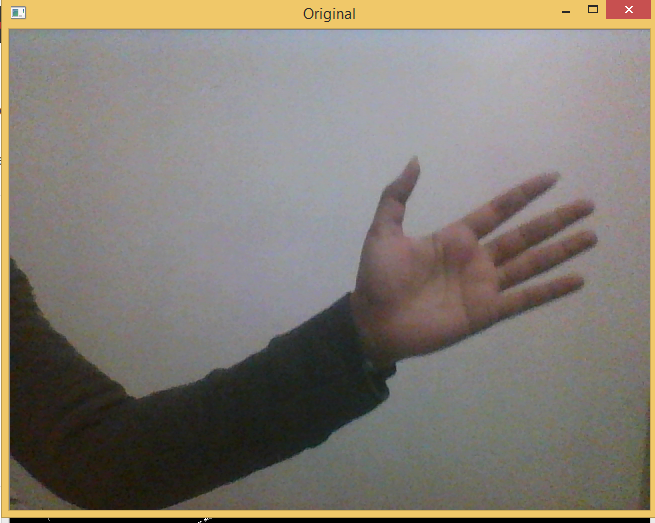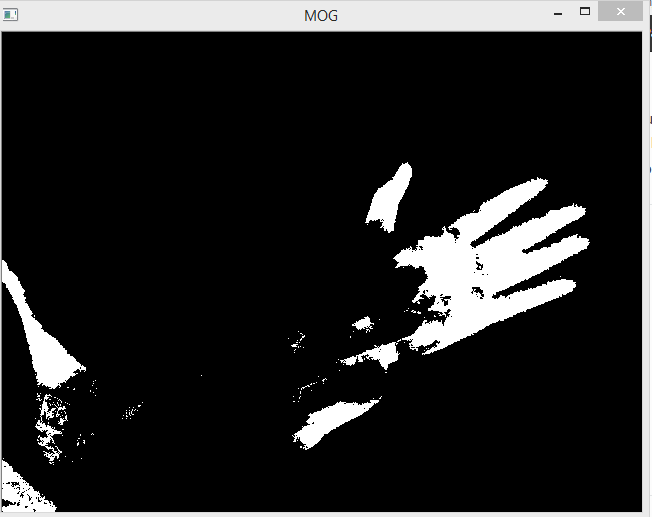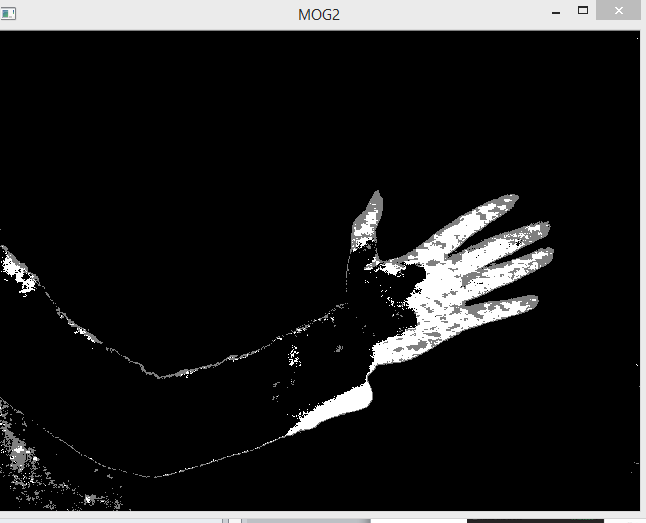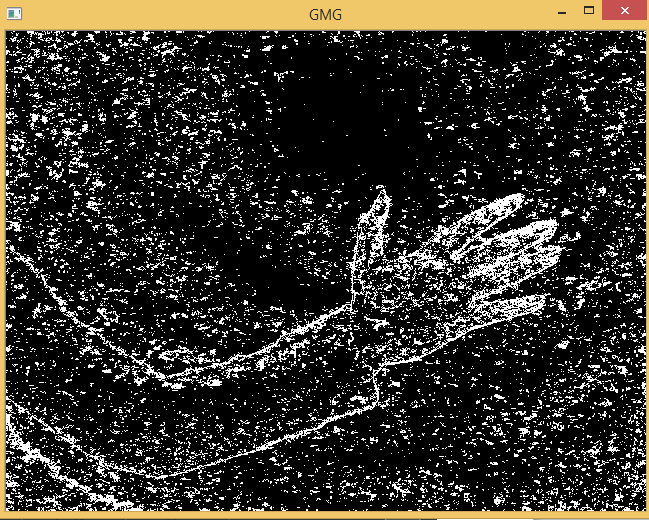We can see that there is a lot of noise in the resultant image for `BackgroundSubtractorGMG`, hence it is always preferred to use morphological transformation to the result to remove the noises.

 `# importing libraries ` `import` `numpy as np ` `import` `cv2 ` ` `  `kernel ``=` `cv2.getStructuringElement(cv2.MORPH_ELLIPSE, (``3``, ``3``)); ` ` `  `# creating object ` `fgbg ``=` `cv2.bgsegm.createBackgroundSubtractorGMG(); ` ` `  `# capture frames from a camera  ` `cap ``=` `cv2.VideoCapture(``0``); ` `while``(``1``): ` `    ``# read frames ` `    ``ret, img ``=` `cap.read(); ` `     `  `    ``# apply mask for background subtraction ` `    ``fgmask ``=` `fgbg.``apply``(img); ` `     `  `    ``# with noise frame ` `    ``cv2.imshow(``'GMG noise'``, fgmask); ` `     `  `    ``# apply transformation to remove noise ` `    ``fgmask ``=` `cv2.morphologyEx(fgmask, cv2.MORPH_OPEN, kernel); ` `     `  `    ``# after removing noise ` `    ``cv2.imshow(``'GMG'``, fgmask); ` `     `  `    ``k ``=` `cv2.waitKey(``30``) & ``0xff``; ` `    ``if` `k ``=``=` `27``: ` `        ``break``; ` ` `  `cap.release(); ` `cv2.destroyAllWindows(); `

Output: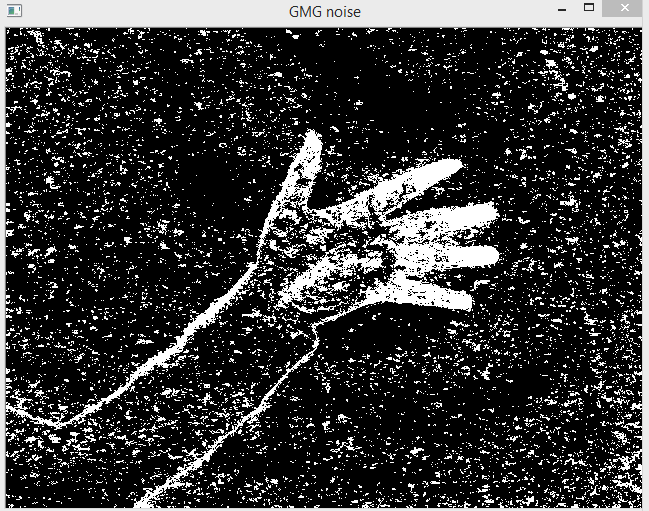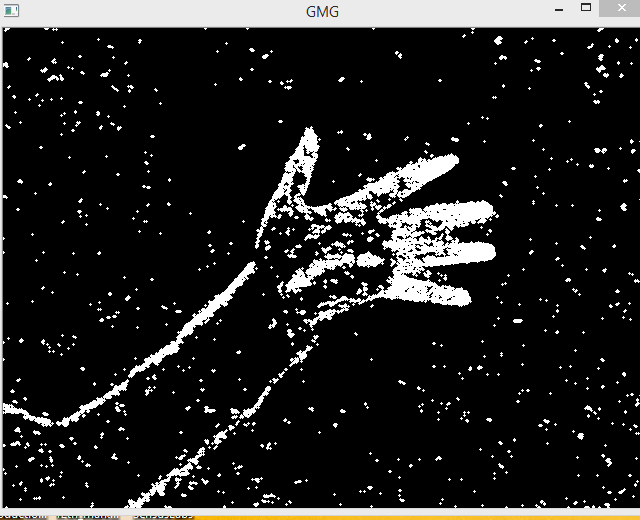My Personal Notes arrow_drop_up
Recommended Articles
Page :